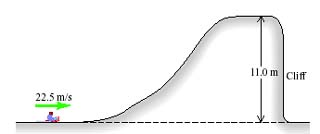# A sled with rider having a combined mass of 120 kg travels over the perfectly smooth icy hill shown in the accompanying figure. How far does the sled land from the foot of the cliff (in m)?sjeikdom0 2021-01-24 Answered

A sled with rider having a combined mass of 120 kg travels over the perfectly smooth icy hill shown in the accompanying figure. How far does the sled land from the foot of the cliff (in m)?You can still ask an expert for help

• Questions are typically answered in as fast as 30 minutes

Solve your problem for the price of one coffee

• Math expert for every subject
• Pay only if we can solve itau4gsf
The rider begins with

and
$PE=mgh=120\cdot 9.8\cdot 0=0$
so,

where
KE=kinetic energy
PE=potential energy
E=total energy=KE+PE
At the top of the hill some of the KE is turned into PE=mgh
PE becomes

and since total energy is conserved KE becomes

Now since the hill is 11.0 m high the period of time of thevertical fall is given by
$h={v}_{0}t+\frac{1}{2}a{t}^{2}$
h=11.0m
${v}_{0}=0$ (this is the initial vertical velocity)
a=9.8m/s
solve for t using the quadratic formula
$t=\frac{-{v}_{a}±\sqrt{{v}_{0}^{2}-4\left(\frac{1}{2}a\right)\left(-h\right)}}{2\left(\frac{1}{2}a\right)}$
$t=±\frac{\sqrt{2ah}}{a}$
choose the positive answer, as the negative answer for time is meaningless

so we know it takes 1.5 sec to hit the ground and we know the horizontal velocity is v=17.05m/s, distance travelled before impact: